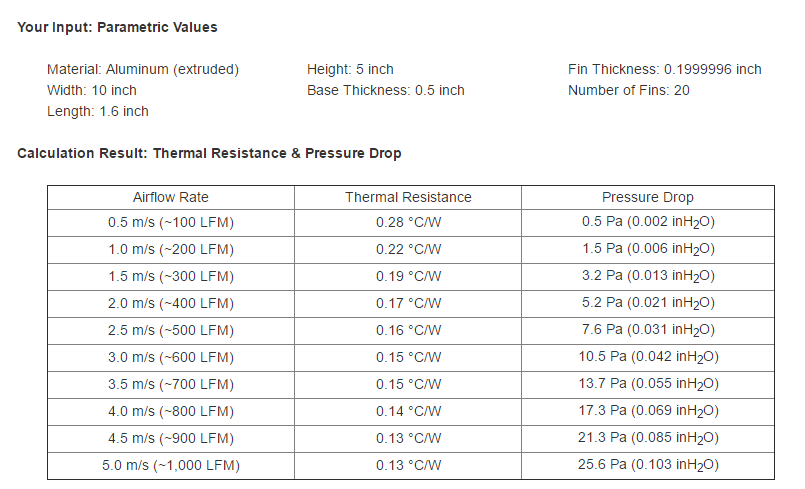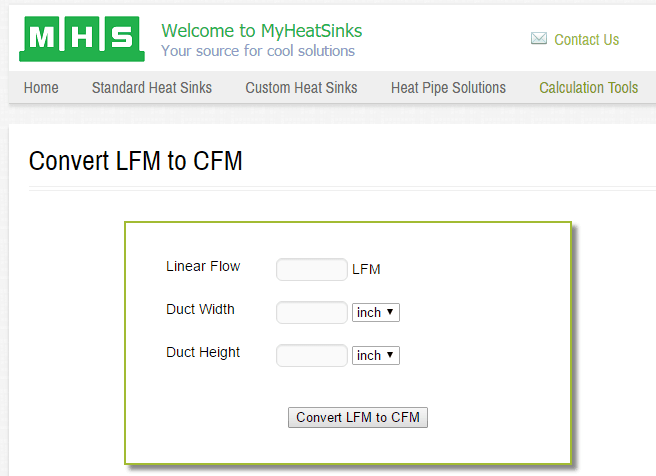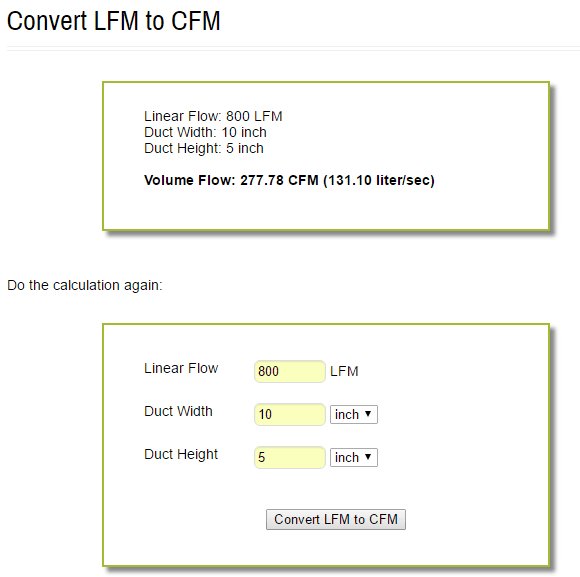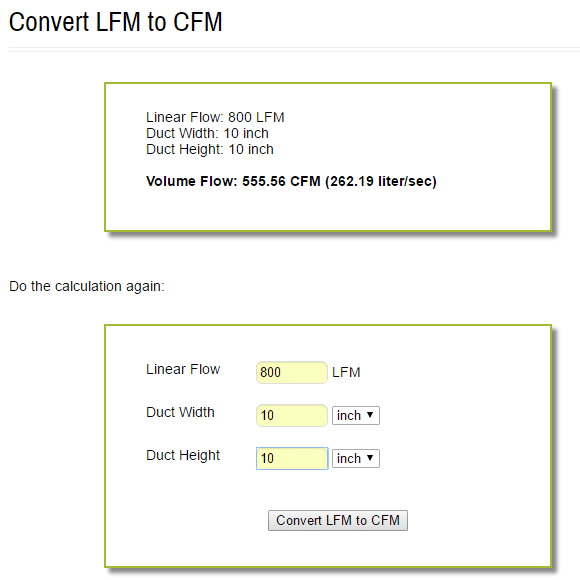# Simple Heat Sink Design Guide for Power Electronics

Heat sink is the soul mate of a power device when talking to power electronics. Thus, right sizing and selection of heat sink is a must. However, an optimized heat sink design will most of the times concluded once there is a working prototypes wherein the thermal profile is available. There are some ways to determine the heat sink size prior to prototype that at least only little fine tuning is needed. In this article we will learn simple heat sink design guide for power electronics and related applications.

## Simple Heat Sink Design Guide

1. Calculate the thermal resistance of the heat sink needed.

Use the tutorial “Heat Sink Thermal Resistance Calculation Easy Explanation” to guide you. If you already have the thermal resistance, you can proceed to next step.

2. Use the online heat sink designer found at http://www.myheatsinks.com or click below link to direct you on the heat sink design section http://www.myheatsinks.com/calculate/thermal-resistance-plate-fin/#

This Simple Heat Sink Design Guide features the tool mentioned above as it is very handy to use though its result is not personally verified by me.

3. Set the parameters found below.

Mind the units. If you want mm then select mm from the drop down list beside each dimension.You are free to input width, length and height. For base thickness, be sure to use a value less than the height to emphasize a fin type design. The number of fins will be limited by the fin thickness and the width. You can do a simple Math to determine the number of fins suited to your dimensions.

4. Once you define the parameters above, click the “Calculate” button.

On the calculation result (below figure), verify if the thermal resistance you calculated is on the table. If not, go back to step number 3 and recalculate until the desired heat sink thermal resistance reflects on the calculation result.5. Interpreting the Calculation Result

Once the calculation result displays the desired thermal resistance, then you got the heat sink dimensions you wanted. However, there is still a trick. The calculation result has 10 rows that correspond to different airflow rate in LFM unit. For instance the thermal resistance I am aiming is 0.14’C/W at 800LFM, what this mean? This mean that I can use the dimensions I specified but with an airflow rate of 800LFM. Fan manufacturers specify fan airflow in terms of CFM. CFM stands for cubic feet per minute. However, when comes to heat sink, LFM is mostly used. LFM stands for linear feet per minute. The relation of CFM and LFM is given by the equation below:

LFM = CFM/Cross-sectional Area

(Note: cross sectional area is in unit square feet)

6. Use online tool to further explain LFMSupposing the thermal resistance 0.14’C/W that corresponds to 800LFM as above, we will find out what should be the CFM of the fan needed and the required length and width of a rectangular duct (let us use a rectangular cross-section in this example).

On the website, type the LFM which is 800 in our example above. Then specify the duct width and height in inches or mm then click “Convert LFM to CFM”. For instance the width is 10 inches while the height is 5 inches, the corresponding CFM is 277.78.So, choose a fan than can give a minimum of 277.78 CFM. If your dimension is larger (supposing 10”X10”) with constant LFM, it requires a higher CFM.Wrapping up things…

From the calculation result, if you intend your thermal resistance needed to fall to lower LFM, say at 200LFM,

basically you need only a fan that has lower CFM rating and this is a smaller fan. However, the heat sink dimensions will be larger.A given CFM will have a lower LFM if the cross-sectional area is large.

“CFM is cubic feet per minute. It describes a volumetric flow of a substance. In the case of a blower or fan, it indicates how much air it can move per minute. A 125cfm fan can remove all of the air from a box that is 5ft x 5ft x 5ft in one minute (assuming vents allow fresh air to fill the vacant space).” Courtesy from Engineering.com.

If you have a simpler way than this Simple Heat Sink Design Guide, you may share it here. Your ideas is very important for us to come up with a more realistic result.

This site uses Akismet to reduce spam. Learn how your comment data is processed.